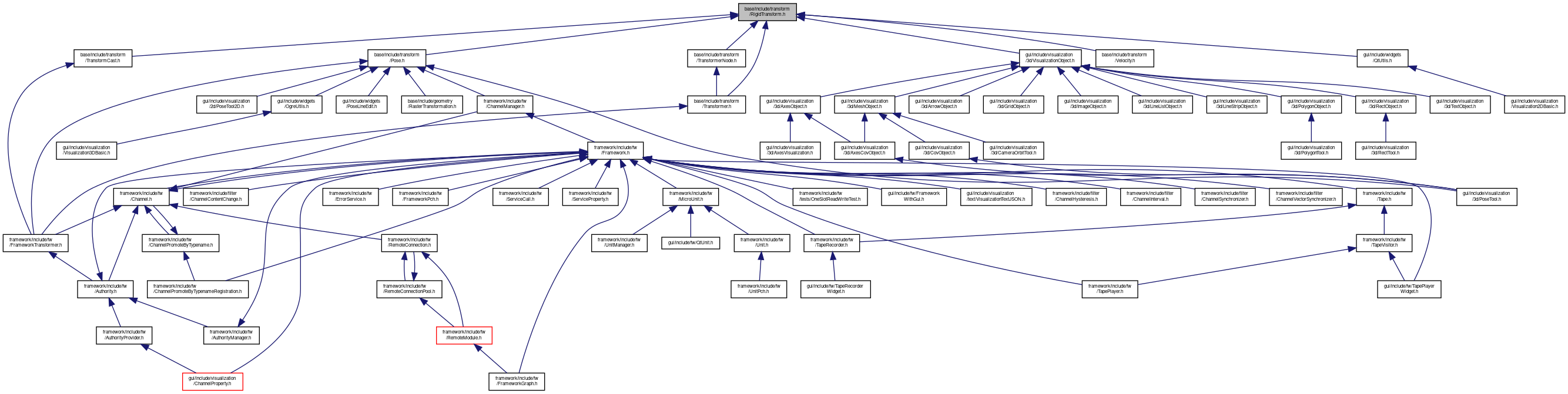MIRA
RigidTransform.h File Reference

Rigid transformation class. More...

`#include <algorithm>`
`#include <limits>`
`#include <math/Angle.h>`
`#include <math/Eigen.h>`
`#include <math/Lerp.h>`
`#include <math/YawPitchRoll.h>`
`#include <serialization/GetterSetter.h>`
`#include <platform/Types.h>`
Include dependency graph for RigidTransform.h:This graph shows which files directly or indirectly include this file:Go to the source code of this file.

## Classes

class  RigidTransform< T, D >
This class represents an affine transformation that supports a translation followed by a rotation (a so called rigid transform). More...

class  RigidTransformCov< T, D >
This class represents an affine transformation that supports a translation followed by a rotation (a so called rigid transform). More...

class  RigidTransformBase< T, D, TRotation, TransformDerived >
Implementation of RigidTransforms with different dimensionality D. More...

class  RigidTransform< T, 2 >
Specialization of RigidTransform for 2 dimensions. More...

class  RigidTransformCov< T, 2 >
Specialization of RigidTransformCov for 2 dimensions. More...

class  RigidTransform< T, 3 >
Specialization of RigidTransform for 3 dimensions. More...

class  RigidTransformCov< T, 3 >
Specialization of RigidTransformCov for 3 dimensions. More...

## Namespaces

mira
specialize cv::DataType for our ImgPixel and inherit from cv::DataType<Vec>

## Typedefs

typedef RigidTransform< float, 2 > RigidTransform2f
Typedef for 2D float transform. More...

typedef RigidTransform< float, 3 > RigidTransform3f
Typedef for 3D float transform. More...

typedef RigidTransform< double, 2 > RigidTransform2d
Typedef for 2D double transform. More...

typedef RigidTransform< double, 3 > RigidTransform3d
Typedef for 3D double transform. More...

typedef RigidTransformCov
< float, 2 >
RigidTransformCov2f
Typedef for 2D float transform with covariance. More...

typedef RigidTransformCov
< float, 3 >
RigidTransformCov3f
Typedef for 3D float transform with covariance. More...

typedef RigidTransformCov
< double, 2 >
RigidTransformCov2d
Typedef for 2D double transform with covariance. More...

typedef RigidTransformCov
< double, 3 >
RigidTransformCov3d
Typedef for 3D double transform with covariance. More...

## Functions

template<typename T , int D, typename S >
RigidTransform< T, D > lerp (const RigidTransform< T, D > &t1, const RigidTransform< T, D > &t2, S alpha)
Linearly interpolates between two transformations and returns the interpolated transform. More...

template<typename T , int D, typename S >
RigidTransformCov< T, D > lerp (const RigidTransformCov< T, D > &t1, const RigidTransformCov< T, D > &t2, S alpha)
Linearly interpolates between two transformations and returns the interpolated transform. More...

## Detailed Description

Rigid transformation class.

Date
2010/09/01